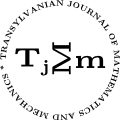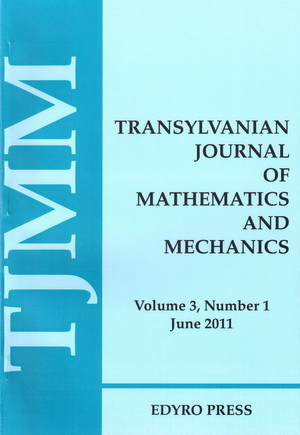ISSN: 2067-239X
ISSN(on-line): 2067-239X

Indexed in:
Mathematical Reviews
Zentralblatt MATH
EBSCO

Front coverVolume 12 (2020), Number 2

New Differential Operator Involving the $q$-Ruscheweyh Derivative and the Symmetric Differential

Author(s): KHALID ALSHAMMARI and MASLINA DARUS

Abstract: In this paper, we define a new differential operator involving $q$-calculus by using a familiar technique which is a Hadamard product. We use convolution between $q$-analogue of the Ruscheweyh derivative by Aldweby and Darus  and the symmetric Salagean differential operator by Ibrahim and Darus . We study some properties of the new operator such as the negative coefficients estimate, growth and the radii of starlikeness and convexity.

A Landau’s Inequality for Semigroups Revisited

Author(s): GEORGE A. ANASTASSIOU

Abstract: H. Kraljevič and S. Kurepa in 1970 () proved a nice Landau type inequality for semigroups by making use of Taylor’s formula with integral remainder for semigroups, which has been a traditional method for proving such inequalities. The author offers another method in the semigroups and reproves the above result by using his earlier result about Ostrowski inequalities for semigroups, see , Chapter 16, pp. 259-289. He finds his method easier and convenient. An application is given at the end.

Positive Solutions to Caputo-Hadamard Fractional Integro-Differential Equations With Integral Boundary Conditions

Author(s): ABDELOUAHEB ARDJOUNI

Abstract: In this article, we study the existence and uniqueness of positive solutions of a Caputo-Hadamard fractional integro-differential equation with integral boundary conditions. The fixed point theorems and the method of upper and lower solutions are used to obtain the desired results. An example illustrating the main results is presented.

An Integral Equation From Physics - a Synthesis Survey - Part III

Author(s): MARIA DOBRIȚOIU

Abstract: This is the third part of the synthesis survey on the study of the integral equation from physics: $x(t)= ∫ab K( t,s, x(s),x( a),x(b) )ds+ f( t), t∈[ a,b ],$ and contains the results concerning the approximation of its solution by applying numerical methods. This part ends with an example.

Some Integral Inequalities for Operator Monotone Functions

Author(s): SILVESTRU SEVER DRAGOMIR

Abstract: In this paper we prove among others that $∫ Tptf( At)At dμ(t) ⩾∫ Tpsf( As)dμ (s) ∫Tpt Atdμ(t )$ for an operator monotone function $f$ on $(0,∞)$, where $( At)t ∈T$ is a bounded continuous field of positive operators in $B ( H )$ defined on a locally compact Hausdorff space $f$ with a bounded Radon measure $μ$ that satisfy certain conditions while $(pt )t∈T$ are nonnegative with $∫T ptdμ (t)=1$. Some particular inequalities of interest are also provided.

Characterizations of Strongly Generalized Convex Functions

Author(s): MUHAMMAD ASLAM NOOR and KHALIDA INAYAT NOOR

Abstract: In this paper, we define and consider some new concepts of the strongly general convex functions with respect to two arbitrary functions. Some properties of the strongly generalized convex functions are investigated under suitable conditions. It is shown that the optimality conditions of the strongly generalized convex functions are characterized by a class of variational inequalities, which is called the strongly generalized variational inequality. Some special cases also discussed. Results obtained in this paper can be viewed as refinement and improvement of previously known results.

On the Generalized Ostrowski Type Inequalities via Tempered Fractional Integrals

Author(s): MEHMET ZEKI SARIKAYA and MEHMET EYÜP Kİ̇Rİ̇Ş

Abstract: In this paper, we have found a new version of the Ostrowski type inequality for tempered Riemann-Liouville fractional integrals. In addition, some relevant results have been obtained.